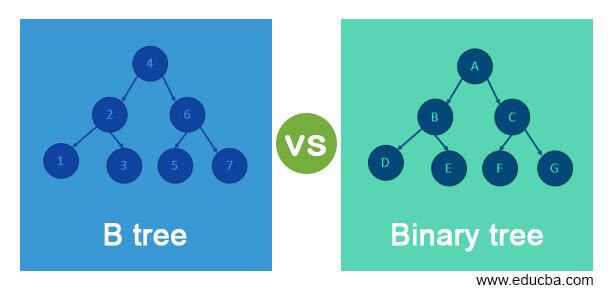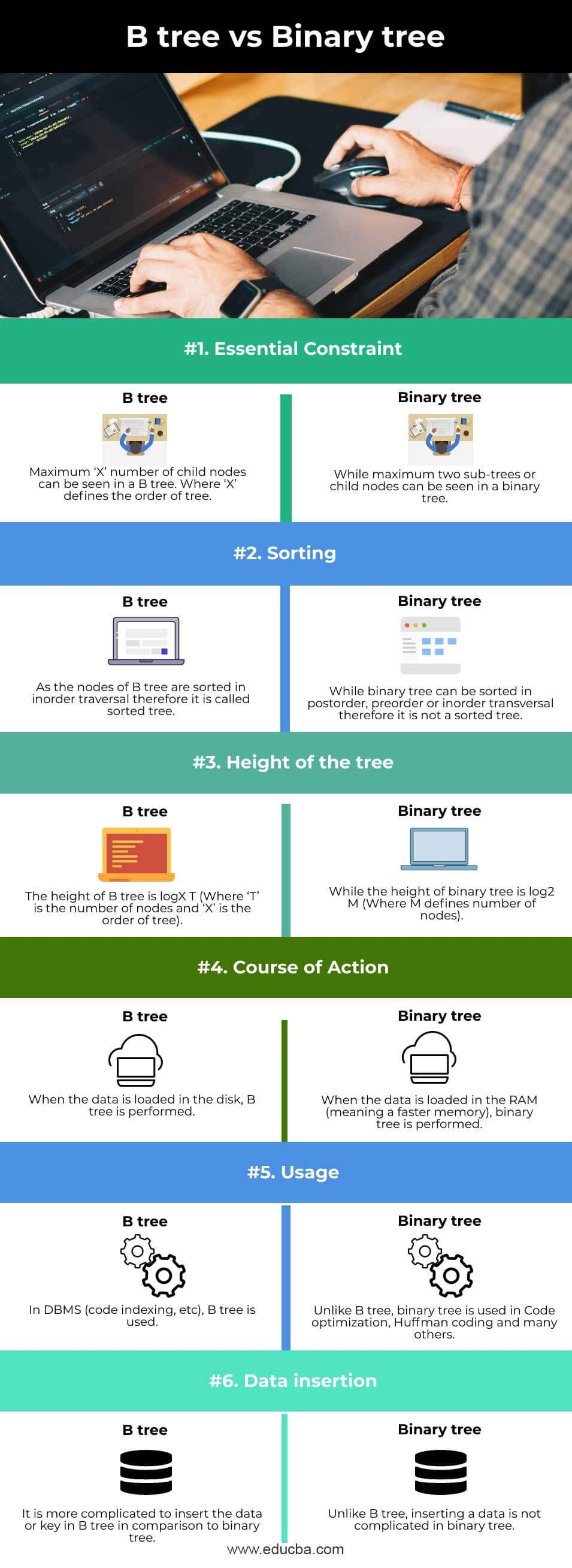# B tree vs Binary tree | Top Differences of B Tree vs Binary Tree

21## Difference between B tree vs Binary tree

On account of its inorder transversal, B-Tree is called a self-balancing search tree. We can also say that a B-tree is the balanced N-way tree and also the balanced sort tree. B-tree is similar to binary search tree but in B-tree, the nodes are organized on the basis of inorder traversal. B-tree has O(n) as the space complexity and O (log n) is the insertion and deletion time complexity in B-tree.

Binary tree is a non-linear data structure and a special general tree. At most two pointers are held by the binary tree for its child nodes. This means that a node can have is 2 as the highest degree and there could be a one-degree node or zero node too.

### Head to Head Comparison Between B tree vs Binary tree (Infographics)

Below are the top 6 differences between B tree vs Binary tree:### Comparison Table of B Tree vs Binary Tree

Let’s look at the comparisons of both in the table below.

 S.No. Description B tree Binary tree 1 Essential Constraint Maximum ‘X’ number of child nodes can be seen in a B tree. Where ‘X’ defines the order of the tree. While a maximum of two sub-trees or child nodes can be seen in a binary tree. 2 Sorting As the nodes of the B tree are sorted in inorder traversal therefore it is called a sorted tree. While a binary tree can be sorted in postorder, preorder, or in order transversal, therefore, it is not a sorted tree. 3 Height of the tree The height of the B tree is logX T (Where ‘T’ is the number of nodes and ‘X’ is the order of the tree). While the height of the binary tree is log2 M (Where M defines a number of nodes). 4 Course of Action When the data is loaded in the disk, a B tree is performed. When the data is loaded in the RAM (meaning a faster memory), a binary tree is performed. 5 Usage In DBMS (code indexing, etc), a B tree is used. Unlike B tree, a binary tree is used in Code optimization, Huffman coding, and many others. 6 Data insertion It is more complicated to insert the data or key in the B tree in comparison to the binary tree. Unlike B tree, inserting data is not complicated in a binary tree.

### Key Differences between B tree and Binary tree

• B Tree

Unlike a binary tree, a node of a B tree can have more than two children. logX T is considered as the height of B tree (Where ‘T’ is the number of nodes and ‘X’ is the order of tree). With each update, the height of B tree is adjusted automatically. The data in B tree is sorted in a specific order, where the highest value on the right and the lowest value on the left. If we want to insert the key or data in B tree, it would be more complicated as compared to the binary tree.

There are some conditions, which are very important to be held by the B-Tree:

1. Every leaf node of the B tree should be at the same level.
2. There should be no empty sub-trees, above the leaf nodes of the B tree.
3. The height of the B tree must be kept as low as possible.
4. Except leave nodes, all nodes of the B tree should have the least number of children.

B tree of order M has these properties:

1. Each and every node of the tree can consist of at most N children and should have at least N/2 or more than 2 to the highest limit.
2. The maximum number of keys that every node can have is N-1 if total children are N or it can also be said as the total keys should be one less than the total children the tree has.
3. Within the nodes, the keys of the B tree are arranged in a specified order. Amongst the keys of the subtree, which are present on the right side are known as successors and eventually the left side residing keys are considered predecessors.
4. While inserting a complete node, the tree is bifurcated into two parts. The key which eventually gets the median value is put as the parent node.
5. The process of merging can be done once the nodes get deleted.

• Binary Tree

Each node can have at most two children in a binary tree and it is a tree data structure. These two children of the binary tree are usually identified as the right child and the left child. A leaf node is defined as a node without children. On account of this, each node in a binary tree can have either 0, 1, or 2 children. Insertion, traversal, and deletion are the common types of operations performed on binary trees. Each node of a tree is visited exactly once in a systematic way in the transversal operation.

Based on the properties of the binary tree, it can be divided into various variants including complete, threaded, extended, strictly, and many other binary trees.

• Strictly Binary tree – This type of tree has a right and left subtree for each and every non-terminal.
• Completely Binary tree – This type of tress satisfies 2 Nodes in each and every node condition. In this, i is considered the level.
• Extended Binary tree – is defined as a type of binary tree where all the null subtree of the original tree are replaced with special nodes known as external nodes whereas other nodes are known as an internal node
• Threaded binary – to make inorder traversal faster and do it without recursion and without stack, is the idea of threaded binary trees. By making all-right child pointers that would normally be a NULL point to the inorder successor of the node (if it exists), we can make a binary tree a threaded binary tree. A threaded binary tree consists of either 0 no of nodes or 2 number of nodes.

### Conclusion

On the basis of the above discussion, we got to know about the B tree and binary tree. We also understood the key differences between B tree and binary tree through the comparison table as well as detailed discussion. We also discussed the different types of Binary trees on the basis of their different properties.

### Recommended Articles

This is a guide to B tree vs Binary tree. Here we discuss the B tree vs Binary tree key differences with infographics and comparison table, respectively. You may also have a look at the following articles to learn more –

The post B tree vs Binary tree appeared first on EDUCBA.

Source linkFounder OF Postintrend.com started my blogging journey in 2015 In This Duration I learned more about blogging, SEO, SEM, Affiliate marketing and more kinds of stuff on the Internet. I love to share my information with everyone.